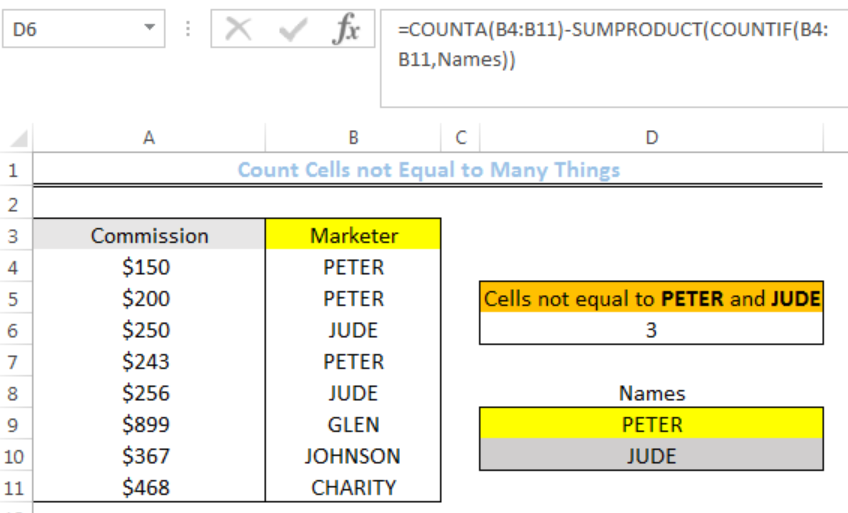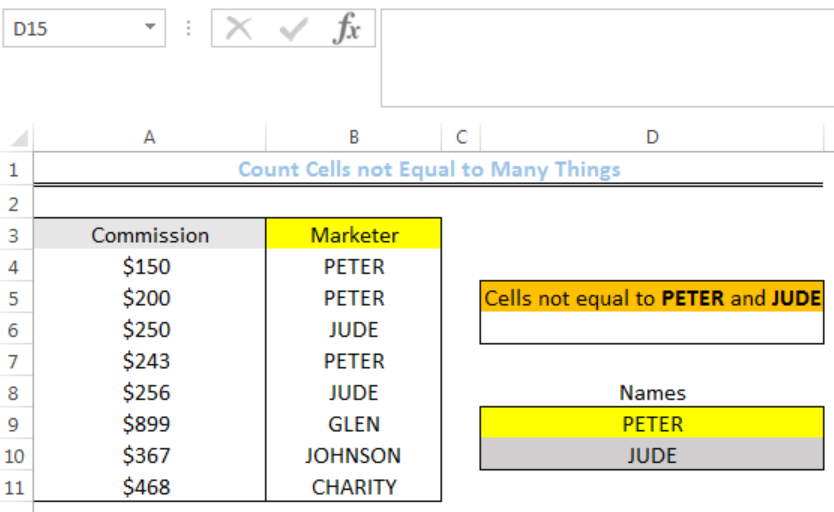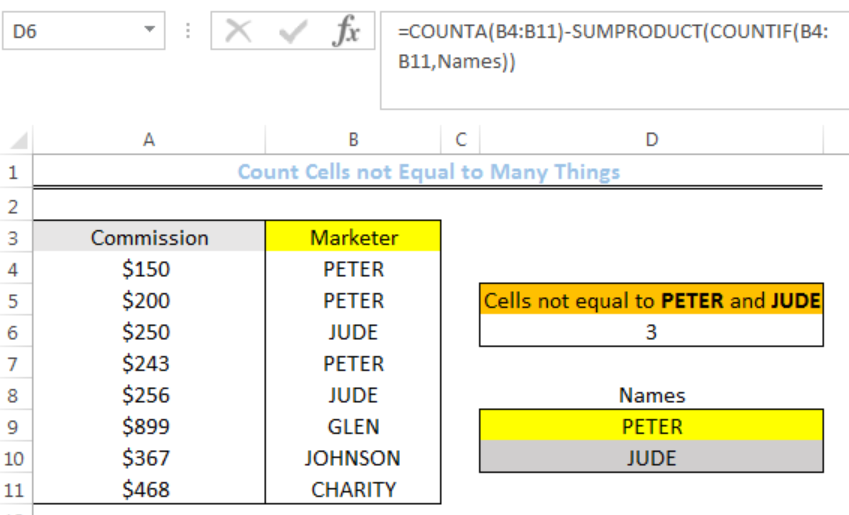Get instant live expert help with Excel or Google Sheets“My Excelchat expert helped me in less than 20 minutes, saving me what would have been 5 hours of work!”

#### Post your problem and you’ll get Expert help in seconds.

Your message must be at least 40 characters
Our professional Expert are available now. Your privacy is guaranteed.

# How to Count Cells Not Equal to Many Things

We can count cells not equal to many things with a combined formula that utilizes the COUNTIF, SUMPRODUCT, and COUNTA functions. This is useful to decipher cells that do not belong to the same category. The steps below will guide all levels of excel users to count cells not equal to many things.Figure 1: Result for Cells not Containing PETER and JUDE

## General formula

`=COUNTA(range)-SUMPRODUCT(COUNTIF(range, things))`

## Formula

`=COUNTA(B4:B11)-SUMPRODUCT(COUNTIF(B4:B11,Names))`

## Setting up the Data

• We will set up the data by inputting the commission for different marketers in Column A and the names of the marketers in Column B
• Cell D6 is where we want the formula to return the result for cells that are not equal to PETER and JUDE
• It should be noted that we must name Cell D9 and Cell 10 as “Names.” We do this by highlighting both cells and click on where we see D15 in figure 2. We will type Names and press the enter keyFigure 2: Setting up the Data

## Count Cells That Are Not Equal To PETER And JUDE

• We will click on Cell D6 and insert the formula below
`=COUNTA(B4:B11)-SUMPRODUCT(COUNTIF(B4:B11,Names))`
• We will press the enter keyFigure 3: Result for Cells not Containing PETER and JUDE

## Explanation

`=COUNTA(B4:B11)-SUMPRODUCT(COUNTIF(B4:B11,Names))`

The COUNTIF function checks the range B4:B11 for cells that contain PETER and JUDE. It then returns the results as TRUE or FALSE like this:

`{TRUE;TRUE;TRUE;TRUE;TRUE;FALSE;FALSE;FALSE}`

The SUMPRODUCT function reads any TRUE VALUE as 1 and FALSE as 0 in the array form below. It sums up the numbers and the result is 5

`{1;1;1;1;1;0;0;0}`

The COUNTA function counts all the cells in the range B4:B11. The total number of cells in the range is 8.

Hence, `8-5=3`.

## Instant Connection to an Expert through our Excelchat Service

Most of the time, the problem you will need to solve will be more complex than a simple application of a formula or function. If you want to save hours of research and frustration, try our live Excelchat service! Our Excel Experts are available 24/7 to answer any Excel question you may have. We guarantee a connection within 30 seconds and a customized solution within 20 minutes.

Solution examplesI need to count number of dates from a timestamped date in column from the completed date column to show the number of days. I have not successfully been able to do this. I am a novice Excel user. Can you help?
Solved by O. B. in 15 minsi have a table with Dates, First names , Last names and i want to count how many times a name occurs, but if a name occurs more than once on a particular date i need to only count it once. this is on excel and not google sheets
Solved by F. H. in 31 minsUse the Vlookup Function to complete the "employee" column of table 2. Use "job Id" from table 2 as your lookup_value(s) and table 1 as your reference.
Solved by C. H. in 16 minsIf a cell in another sheet is populated I need a vlookup done. If the cell is not populated I need the cell to return blank.
Solved by T. D. in 60 minsI am trying to make a chart that turns a week range red if nothing is entered in the range. If something is entered then I would like it to turn green. Please Help
Solved by E. U. in 43 mins